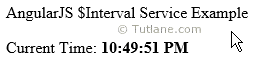# AngularJS Interval Service (\$interval) with Example

Here we will learn what is \$interval service in angularjs, use of \$interval service and how to use \$interval service in angularjs applications to make countdown timer with simple example.

## AngularJS \$interval Service

In angularjs \$interval service is same as window.setInterval function in javascript. By using \$interval service in angularjs we can execute function or method repeatedly with specific time interval based on our requirement.

Suppose we have requirement in angularjs application like execute same function every 1 second. By using \$interval service in angularjs we can achieve this functionality.

## Syntax of AngularJS \$interval Service

Following is the syntax of using \$interval service in angularjs applications.

var app = angular.module('intervalApp', []);

app.controller('intervalCtrl', function (\$scope, \$interval) {

\$scope.ptime = new Date().toLocaleTimeString();

\$interval(function () {

\$scope.ptime = new Date().toLocaleTimeString();

}, 1000);

});

If you observe above syntax we are executing \$interval function every 1 second. Here we defined time in milliseconds (1 sec = 1000 milliseconds).

Note: In above syntax we passed \$interval as argument to the controller in angularjs. To use service in controller we need to pass \$interval as parameter.

We will see simple example of using \$interval service in angularjs application.

## AngularJS \$interval Service Example

Following is the example of using \$interval service in angularjs applications.

<!DOCTYPE html>

<html>

<title>

AngularJs \$interval Service with Example

</title>

<script type="text/javascript">

var app = angular.module('intervalApp', []);

app.controller('intervalCtrl', function (\$scope, \$interval) {

\$scope.ptime = new Date().toLocaleTimeString();

\$interval(function () {

\$scope.ptime = new Date().toLocaleTimeString();

}, 1000);

});

</script>

<body>

<div ng-app="intervalApp" ng-controller="intervalCtrl">

<p>AngularJS \$Interval Service Example</p>

Current Time: <b>{{ptime}}</b>

</div>

</body>

</html>

Now we will run and see the output of above angularjs \$interval service application.

## Output of AngularJS \$interval Service Example

Following is the result of using \$interval service in angularjs applications.